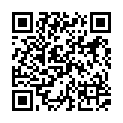# North Coast Synthesis Ltd.

## A♭♭5+2+4+♯1Choose audio sample set:
MSK 007 Leapfrog VCF (patch; see in online store)
MSK 009 Coiler VCF (patch; see in online store)

Equivalent chord symbols: A4+♯1+♯2+♯7, A4+♯1+♯2+♭1, A4+♯1+♯7+♯9, A♭♭5+2+4+♭2, A♭♭5+2+4+♭9.

Notes in this chord: A♭, A, B♭, C, D♭, E♭♭. Integer notation: {0, 1, 2, 8, 9, 10}.

Nearby chords (one less note): A4+♯1+♯2, A4+♯1+♯7, A♭4+2+♯1, A♭♭5+2+4, A♭♭5+2+♯1, A♭♭5+4+♯1.

Nearby chords (one more note): AM11♯9+♯1, A♭11♭5+♯1, B♭9+♯2+♯7, A♭M11♭5+♯1, A♭+2+4+♯1+♯4, A♭dim+2+4+♯1+♯3.

Parallel chords (same structure, different root): C♭♭5+2+4+♯1, D♭♭5+2+4+♯1, E♭♭5+2+4+♯1, F♭♭5+2+4+♯1, G♭♭5+2+4+♯1, B♭♭5+2+4+♯1, E♯♭5+2+4+♯1, B♯♭5+2+4+♯1.

Experimental fretting charts for guitar standard EADGBE tuning (change tuning or instrument):